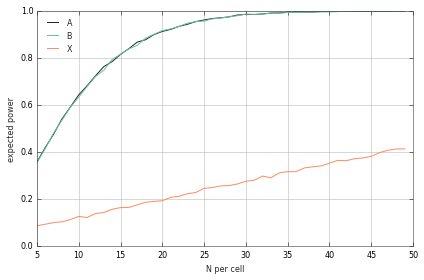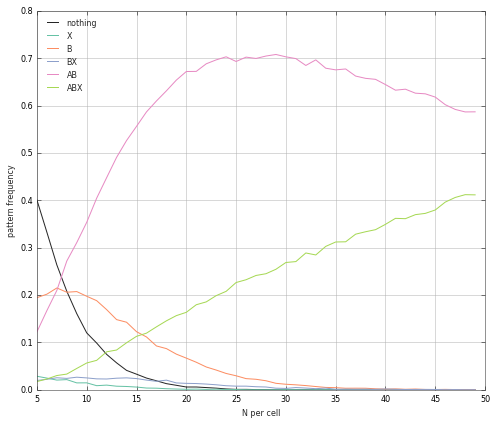# Mozgostroje

## No Way Anova - Interactions need more power

20 Apr 2014

[]

I quote myself from the last post:

The number of tests and the probability to obtain at least one significant result increases with the number of variables (plus interactions) included in the Anova. According to Maxwell (2004) this may be a reason for prevalence of underpowered Anova studies. Researchers target some significant result by default, instead of planning sample size that would provide enough power so that all effects can be reliably discovered.

Maxwell (2004, p. 149) writes:

a researcher who designs a 2 $\times$ 2 study with 10 participants per cell has a 71% chance of obtaining at least one statistically significant result if the three effects he or she tests all reflect medium effect sizes. Of course, in reality, some effects will often be smaller and others will be larger, but the general point here is that the probability of being able to find something statistically significant and thus potentially publishable may be adequate while at the same time the probability associated with any specific test may be much lower. Thus, from the perspective of a researcher who aspires to obtain at least one statistically significant result, 10 participants per cell may be sufficient, despite the fact that a methodological evaluation would declare the study to be underpowered because the power for any single hypothesis is only .35.

What motivates the researcher to keep the N small? Clearly, testing more subjects is costly. But I think that in Anova designs there is additional motivation to keep N small. If we use large N we obtain all main effects and all interactions significant. This is usually not desirable because some of the effects/interactions are not predicted by researcher's theory and non-significant main effect/interaction is taken as an evidence for a lack of this component. Then the researcher needs to find some N that balances between something significant and everything significant. In particular the prediction of significant main effects and non significant interaction is attractive because it is much easier to achieve than other patterns.

Let's look at the probability of obtaining significant main effects and interaction in Anova. I'm lazy so instead of deriving closed-form results I use simulation. Let's assume 2 $\times$ 2 Anova design where the continuous outcome is given by $y= x_1 + x_2 + x_1 x_2 +\epsilon$ with $\epsilon \sim \mathcal{N}(0,2)$ and $x_1 \in \{0,1\}$ and $x_2 \in \{0,1\}$. We give equal weight to all three terms to give them equal start. It is plausible to include all three terms, because with psychological variables everything is correlated (CRUD factor). Let's first show that the interaction requires larger sample size than the main effects.

In :
%pylab inline
from scipy import stats
Ns=np.arange(20,200,4);
K=10000;
ps=np.zeros((Ns.size,3))
res=np.zeros(4)
cs=np.zeros((Ns.size,8))
i=0
for N in Ns:
for k in range(K):
x1=np.zeros(N);x1[N/2:]=1
x2=np.mod(range(N),2)
y= 42+x1+x2+x1*x2+np.random.randn(N)*2
tot=np.square(y-y.mean()).sum()

x=np.ones((N,4))
x[:,1]=x1*x2
x[:,2]=x1*(1-x2)
x[:,3]=(1-x1)*x2
res=np.linalg.lstsq(x,y)

x=np.ones((N,2))
x[:,1]=x1
res=tot-np.linalg.lstsq(x,y)

x[:,1]=x2
res=tot-np.linalg.lstsq(x,y)

res=tot-res-res-res

mss=res/np.float32(np.array([N-4,1,1,1]))
F=mss[1:]/mss
p=1-stats.f.cdf(F,1,N-4)
p=p<0.05
ps[i,:]+=np.int32(p)
cs[i,p*4+p*2+p]+=1
i+=1
ps/=float(K)
cs/=float(K)
for k in range(ps.shape): plt.plot(Ns/4, ps[:,k])
plt.legend(['A','B','X'],loc=2)
plt.xlabel('N per cell')
plt.ylabel('expected power');

Populating the interactive namespace from numpy and matplotlibNow we look at the probability that the various configurations of significant and non-significant results will be obtained.

In :
plt.figure(figsize=(7,6))
for k in [0,1,2,3,6,7]: plt.plot(Ns/4, cs[:,k])
plt.legend(['nothing','X','B','BX','AB','ABX'],loc=2)
plt.xlabel('N per cell')
plt.ylabel('pattern frequency');To keep the figure from too much clutter I omitted A and AX which is due to symmetry identical to B and BX. By A I mean "main effect A is significant and main effect B plus the interaction are not significant". X designates the presence of a significant interaction.

To state the unsurprising results first, if we decrease the sample size we are more likely to obtain no significant result. If we increase the sample size we are more likely to obtain the true model ABX. Because interaction requires large sample size to reach significance for medium sample size AB is more likely than the true model ABX. Furthermore, funny things happen if we make main effects the exclusive focus of our hypothesis. In the cases A,B and AB we can find a small-to-medium sample size that is optimal if we want to get our hypothesis significant. All this can be (unconsciously) exploited by researchers to provide more power for their favored pattern.

It is not difficult to see the applications. We could look up the frequency of various patterns in the psychological literature. This could be done in terms of the reported findings but also in terms of the reported hypotheses. We can also ask whether the reported sample size correlates with the optimal sample size.

Note, that there is nothing wrong with Anova. The purpose of Anova is NOT to provide a test for composite hypotheses such as X, AB or ABX. Rather it helps us discover sources of variability that can then be subjected to a more focused analysis. Anova is an exploratory technique and should not be used for evaluating of hypotheses.